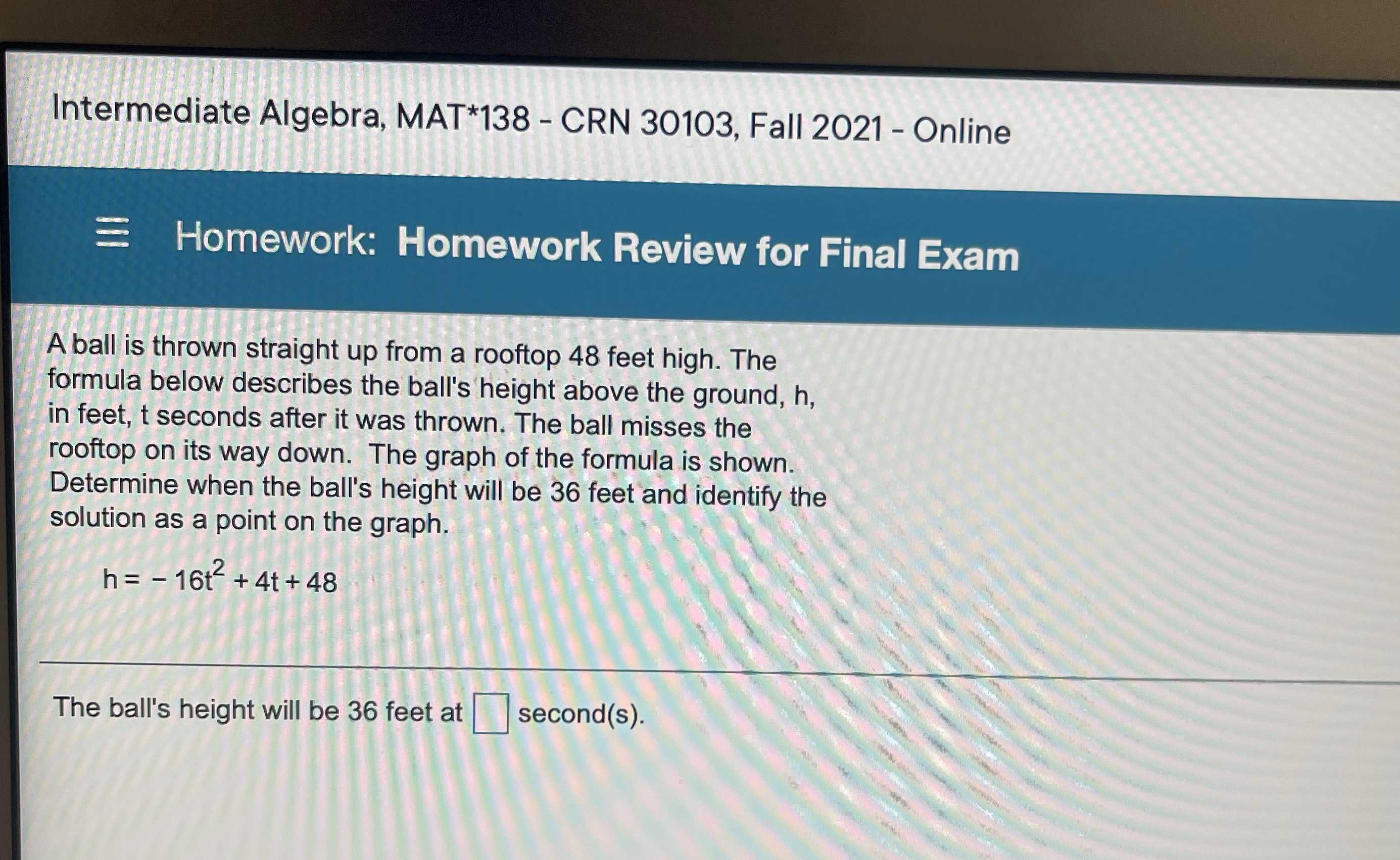### ¿Todavía tienes preguntas de matemáticas?

Pregunte a nuestros tutores expertos
Algebra
PreguntaA ball is thrown straight up from a rooftop $$48$$ feet high. The formula below describes the ball's height above the ground, h, in feet, t seconds after it was thrown. The ball misses the rooftop on its way down. The graph of the formula is shown. Determine when the ball's height will be $$36$$ feet and identify the solution as a point on the graph.

$$h = - 16 t ^ { 2 } + 4 t + 48$$